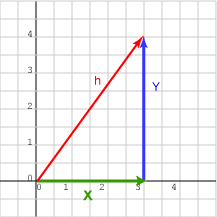What is the length of the hypotenuse of a right triangle whose two sides are 6 and 8?

```length2   =   62 + 82
length2   =   36 + 64 = 100
length    =   10
```

# Formula for Vector LengthThe formula for the length of a 2D vector is the Pythagorean Formula. Say that the vector is represented by   (x, y)T.   Put the vector with its tail at the origin. Now make a triangle by drawing the two sides:

```side_1   = (x, 0)T

side_2   = (0, y)T.
```

The length of side_1 is x, and the length of side_2 is y, so:

```length (x, y)T   =    √( x2 + y2 )
```

In this formula, means the positive square root. We don't (of course) want the length to be negative.

### QUESTION 4:

What is the length of the vector represented by   ( 4, 3 )T     ?